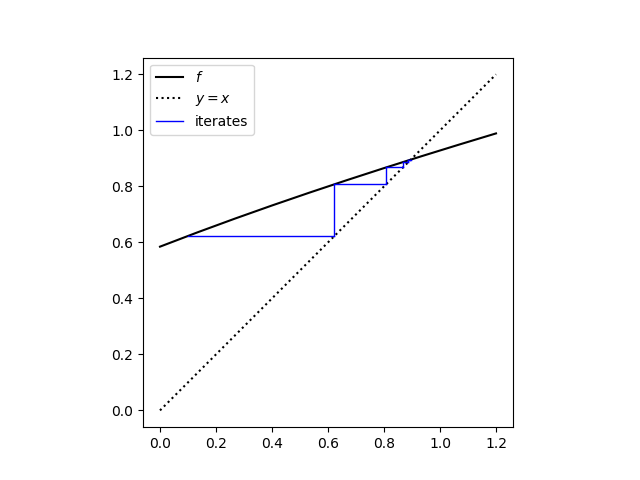# A tale of two iterations

I recently stumbled on a paper  that looks at a cubic equation that comes out of a problem in orbital mechanics:

σx³ = (1 + x

Much of the paper is about the derivation of the equation, but here I’d like to focus on a small part of the paper where the author looks at two ways to go about solving this equation by looking for a fixed point.

If you wanted to isolate x on the left side, you could divide by σ and get

x = ((x + 1)² / σ)1/3.

If you work in the opposite direction, you could start by taking the square root of both sides and get

x = √(σx3) – 1.

Both suggest starting with some guess at x and iterating. There is a unique solution for any σ > 4 and so for our example we’ll fix σ = 5.

We define two functions to iterate, one for each approach above.

```    sigma = 5
x0 = 0.1

def f1(x):
return sigma**(-1/3)*(x+1)**(2/3)

def f2(x):
return (sigma*x**3)*0.5 - 1
```

Here’s what we get when we use the cobweb plot code from another post.

`    cobweb(f1, x0, 10, "ccube1.png", 0, 1.2)`This shows that iterations converge quickly to the solution x = 0.89578.

Now let’s try the same thing for `f2`. When we run

`    cobweb(f2, x0, 10, "ccube2.png", 0, 1.2)`

we get an error message

`    OverflowError: (34, 'Result too large')`

Let’s print out a few values to see what’s going on.

```    x = 0.1
for _ in range(10):
x = f2(x)
print(x)
```

This produces

```    -0.9975
-3.4812968359375005
-106.4783129145318
-3018030.585561691
-6.87243939752166e+19
-8.114705541507359e+59
-1.3358518746543001e+180
```

before aborting with an overflow error. Well, that escalated quickly.

The first iteration converges to the solution for any initial starting point in (0, 1). But the solution is a point of repulsion for the second iteration.

If we started exactly on the solution, the unstable iteration wouldn’t move. But if we start as close to the solution as a computer can represent, the iterations still diverge quickly. When I changed the starting point to 0.895781791526322, the correct root to full floating point precision, the script crashed with an overflow error after 9 iterations.

## More on fixed points

 C. W. Groetsch. A Celestial Cubic. Mathematics Magazine, Vol. 74, No. 2 (Apr., 2001), pp. 145–152.

## 2 thoughts on “A tale of two iterations”

1. I hoped you would throw in :
if r is a root of x = f(x) then
f(x) – r = f(x) – f(r) = f'(c)(x-r) for c between x and r
so estimating |f’| 1 tells you converge or diverge

2. Nathan Hannon

Additionally, since (f^-1)’ = (f’)^-1, you should always expect that one will converge and the other will not, barring some pathology with |f’| = 1.

This is in contrast to the situation with a function of two or more variables, where you can have something like f(x, y) = (x/2, 2y) such that neither f nor f^-1 converges to the fixed point in general.